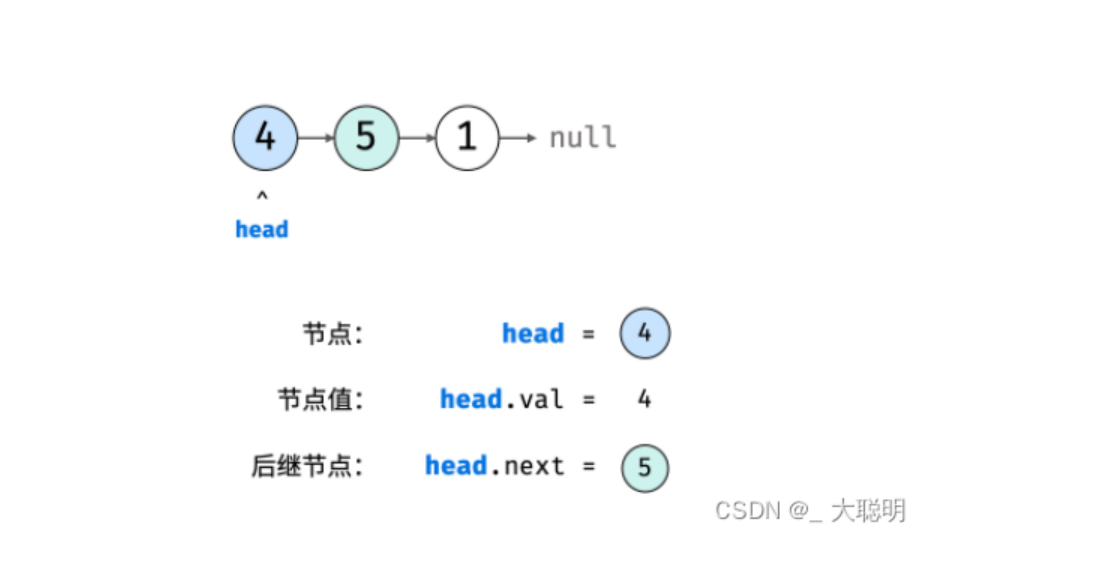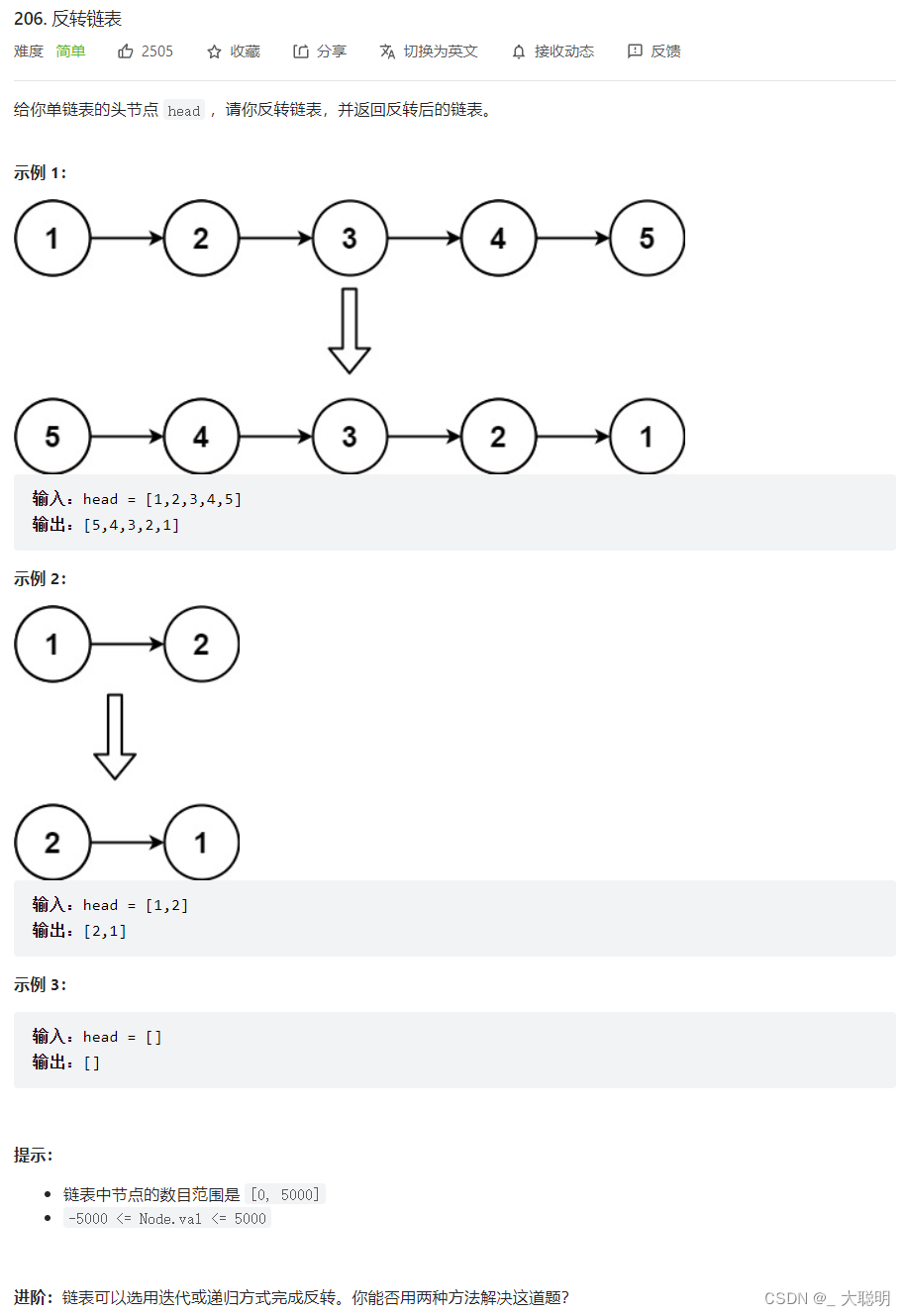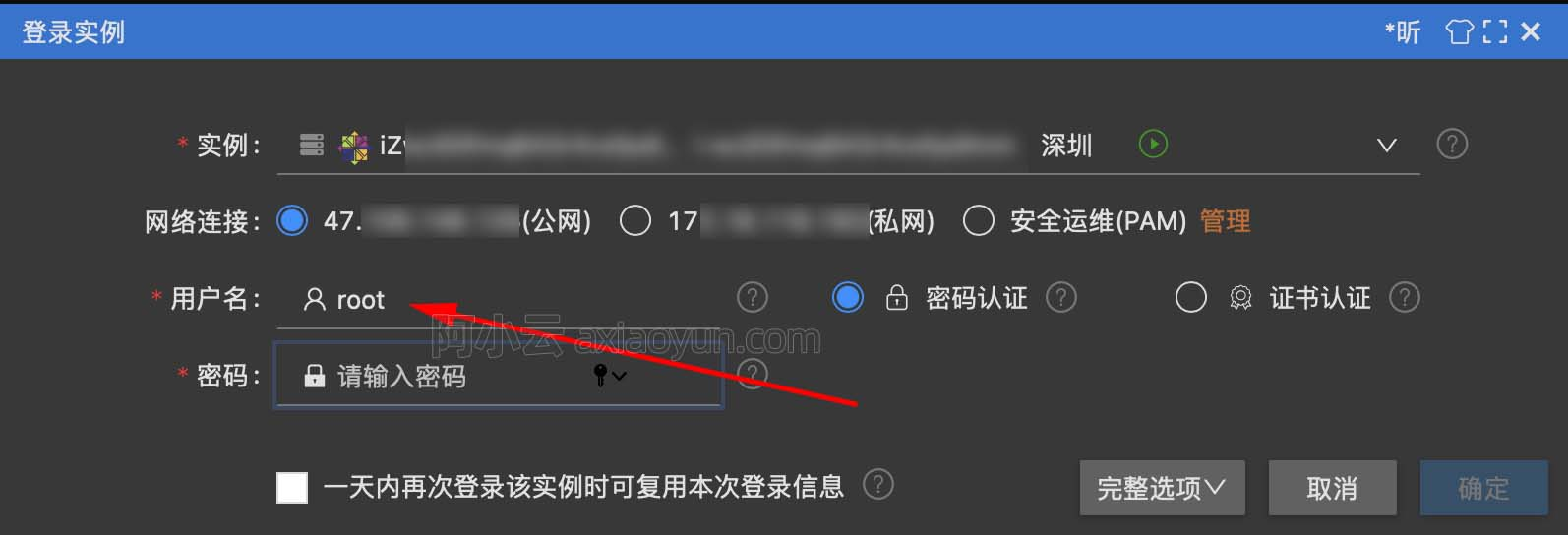# 《Java小子怒闯数据结构九重天》第五重天——链表

+关注继续查看# 1.🌀链表的基础知识

date next

prior date next

## 2.🌀Java实例化链表

class ListNode {
int val;       // 节点值
ListNode next; // 后继节点引用
ListNode(int x) {
val = x;
}
}

// 实例化节点

ListNode n1 = new ListNode(4); // 节点 head
ListNode n2 = new ListNode(5);
ListNode n3 = new ListNode(1);

// 连接节点使之成为链表

n1.next = n2;
n2.next = n3;
n3.next = null;### 3.🌀Java实现单链表

import java.util.Iterator;
/**
* @param
*/
private int N;//结点个数
//构造方法
N = 0;
}

//结点内部类
private class Node{
//存储数据
T item;
//下一个结点
Node next;
public Node(T item,Node next) {
this.item = item;
this.next = next;
}
}

//清空链表
public void clear(){
this.N=0;
}

//判断链表是否为空
public boolean isEmpty(){
return this.N==0;
}

//获取链表的长度
public int length(){
return this.N;
}

//读取链表第i位置的元素值并返回
public T get(int i){
//非法性检查
if(i<0 || i > this.N){
throw new RuntimeException("位置不合法");
}
// n也是指向头结点
for(int index=0; index
n = n.next;
}
return n.item;
}

//往链表中插入数据t
public void insert(T t){
// 获取尾节点
while(true){
// 当刚好就一个节点时（头节点）
if(n.next == null){
break;
}
n = n.next;
}
//当为空表时，就可以插入
Node node = new Node(t, null);
n.next =node;
this.N ++;
}
//在第i位置上插入数据t
public void insert(T t,int i){
// 非法性检查
if(i < 0 || i > this.N){
throw  new RuntimeException("插入位置非法");
}
for(int index=0;index <= i-1; index++){
pre = pre.next;
}
Node current = pre.next;
//先链接后面结点
Node newNode = new Node(t,null);
pre.next = newNode;
newNode.next = current;
this.N++;
}

//移除并返回第i位置的元素值
public  T remove(int i){
// 非法性检查
if(i < 0 || i >this.N){
throw  new RuntimeException("删除位置有误");
}
for(int index=0;index <= i-1;index ++){
n = n.next;
}
//要删除的节点
Node curr = n.next;
n.next = curr.next;
this.N--;//结点个数减一
return curr.item;
}

//查找元素t在链表中第一次出现的位置
public int indexof(T t){
for(int i = 0; n.next != null;i++){
n =n.next;
if(n.item.equals(t)){
return i;
}
}
return -1;
}
@Override
public Iterator iterator() {
return new Iterator() {
@Override
public boolean hasNext() {
return n.next !=null;
}
@Override
public Object next() {
//下移一个指针
n = n.next;
return n.item;
}
};
}
}

4.🌀链表进阶练习

Leetcode 206. 反转链表class Solution {
//申请节点，pre和 cur，pre指向null
ListNode pre = null;
ListNode tmp = null;
while(cur!=null) {
//记录当前节点的下一个节点
tmp = cur.next;
//然后将当前节点指向pre
cur.next = pre;
//pre和cur节点都前进一位
pre = cur;
cur = tmp;
}
return pre;
}
}15880 0Xshell使用SSH远程登录阿里云ECS服务器CentOS7
21692 014852 015291 018581 036339 019980 023736 027727 016589 0
72

0

JS零基础入门教程（上册）